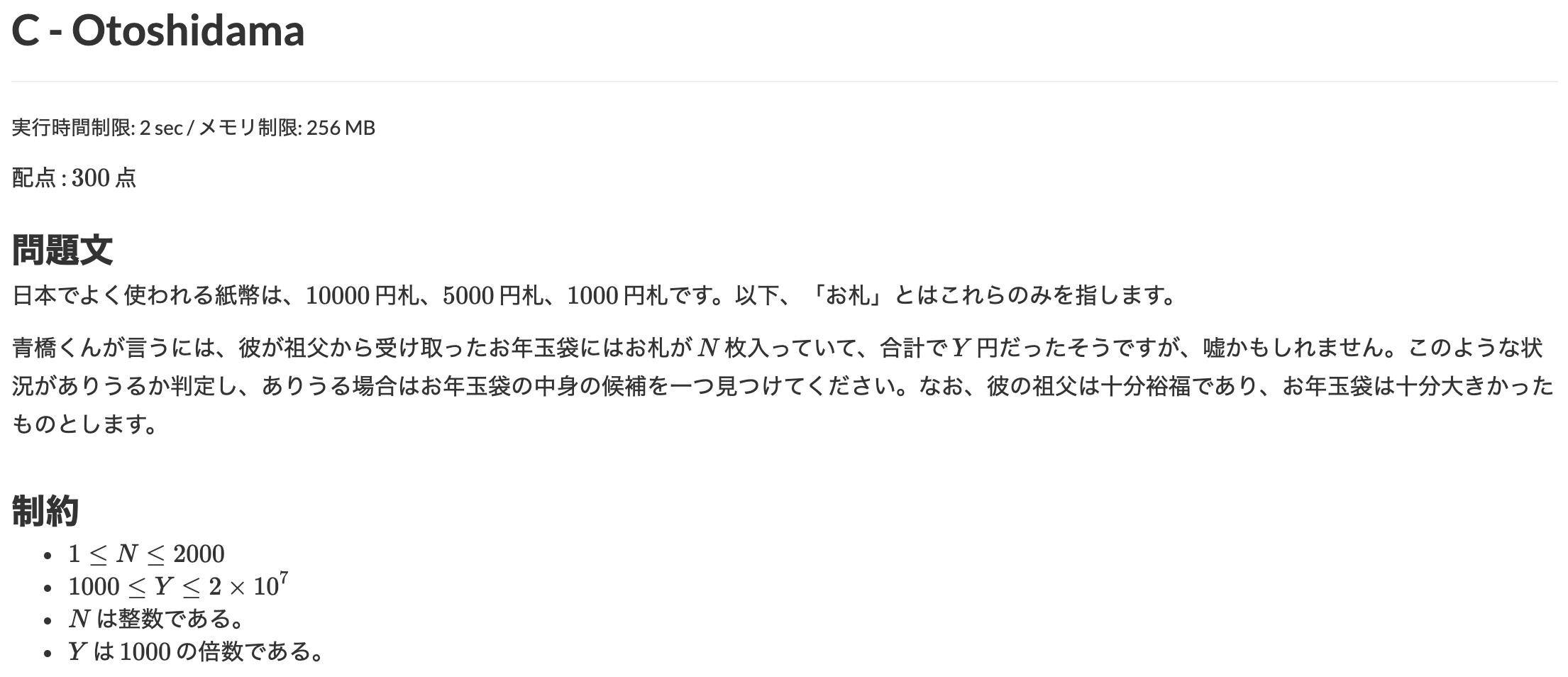# ABC085 C - Otoshidama

atcoder# # Problem

Given 2 integers $N$ and $Y$, report if it's possible to make $Y$ yen with $N$ bills or not.
A bill is either a 10000 yen, a 5000 yen or a 1000 yen.
If it's possible, print any combination of them.

# # Explanation

Letting the numbers of 10000 yen bill, 5000 yen bill and 1000 yen bill $a$, $b$ and $c$, you get 2 equations (1) and (2).
And as $a$, $b$ and $c$ are the number of bills, you get (3).

$\begin{cases} a + b + c = N (1) \\ 10000a + 5000b + 1000c = Y (2) \\ 0 \leq a, b, c \leq N (3) \end{cases}$

From (1) $c$ is unique for a combination of $a$ and $b$.

$c = N - a - b$

If you find any combination of $a$, $b$ and $c$ which satisfies (2) and (3), print it and done.

# # Time complexity

$O(N^2)$

Double loop for making combinations of $a$ and $b$.

# # Solution

#define MAX_N 2001
#define MAX_Y 20000001

Int N, Y;

void input() {
cin >> N >> Y;
}

void solve() {
loop(a,0,N+1) {
loop(b,0,N+1) {
Int c = N - a - b;
if (a + b > N || c < 0) continue;
if (a * 10000 + b * 5000 + c * 1000 == Y) {
cout << a << ' ' << b << ' ' << c << endl;
return;
}
}
}
cout << "-1 -1 -1" << endl;
}

int main(void) {
input();
solve();
return 0;
}Remote freelancer. A web and mobile application enginner.
Traveling around the world based on East Asia.
I'm looking forward to your job offers from all over the world!

Offer jobs or contact me!